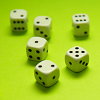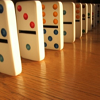#### You may also like### Consecutive Numbers

An investigation involving adding and subtracting sets of consecutive numbers. Lots to find out, lots to explore.### Roll These Dice

Roll two red dice and a green dice. Add the two numbers on the red dice and take away the number on the green. What are all the different possible answers?### Domino Square

Use the 'double-3 down' dominoes to make a square so that each side has eight dots.

# What Do You Need?

##### Age 7 to 11Challenge Level

We received many, many solutions to this What Do You Need? problem, but not so many of you explained how you came to the solution and which clues were useful and which weren't.

Just to warn you - the clues are ordered slightly differently on the printable sheet compared with in the problem itself so do read the solutions below carefully!

Holly from Sacred Heart School in New Zealand told us:

* statement 1 does not help because by following the other clues you can tell that you need more than 1 digit to find the answer and the only multiple of 7 smaller than 9 is 7, which consists of only 1 digit.
* statement 2 does not help because the ones digit has to be larger than the tens digit and the only multiple of 7 and 10 is 70 and the 0 is smaller than the 7
* statement 3 helps because being a multiple of 7 cancels out a lot of numbers that could have been possibilities.
* statement 4 helps because by being an odd number it too cancels out a lot of other possibilities
* statement 5 does not help because the only multiple of 7 and 11 is 77 and the ones digit has to be bigger than the tens digit and the two digits in 77 are even (even - meaning the same, I think) .
* statement 6 does not help because you can only choose the numbers from 1-100, and those numbers are all below 200 anyway, so that statement is completely worthless.
* statement 7 helps because by using it there will only be a few numbers left to choose from.
* statement 8 helps because by using it you can easily narrow the number down so that there is only one left.
The only number left after using all of the useful clues is 35

Children at St Faith's School worked together and sent us the following solution

The rules you need (in order) are:
- The number is odd (so cross out all the columns of even numbers). This is the most important rule because you can get rid of half of the numbers very quickly.
- The tens digit is odd (so cross out all the rows that have even tens numbers. These are 0-9; 20-29; 40-49; 60-69 and 80-89). We decided that all the numbers in the row 0-9 counted as numbers with an even tens number as the tens numbers in this row all equal zero and if we say that an even number is one which doesn't leave a remainder when you divide by 2 then zero counts as an even number. This rule is important because it helps us cross out 25 more numbers.
- The number is a multiple of 7 (so cross out all numbers that are not in the seven times table). There are now only three numbers left: 35, 77 and 91.
- Its ones digit is larger than its tens digit (so cross out all numbers that have a number in the ones (units) column the same as or less than the number in the tens). This rule lets you cross out two of the remaining three numbers to leave the correct answer.
This leaves the number 35 so 35 is the correct answer.

The rules we didn't need were:
- The number is less than 200: you don't need this rule because all the numbers are less than 200 already so none would get crossed out!
- The number is greater than 9: you don't need this rule because numbers 0-9 have zero tens and all these numbers get crossed out later in the problem when you cross out all the numbers that have an even number in the tens.
- The number is not a multiple of ten: you don't need this rule because you have already crossed out all the multiples of tens when you crossed out all the even numbers.
- The number is not a multiple of 11: you don't need this rule because at the end when there are three numbers left (35, 77 and 91) if you used this rule you would only cross out 77 and still have two numbers left and need to use a 5th rule to choose between them so it is better for the last rule to be 'Its ones digit is larger than its tens digit' as this gets rid of two numbers and leaves the right answer.

Cong also explained clearly how he went about the problem:

The number is 35. The way I worked it out is:
I started with 'The number is a multiple of 7' : With that clue only 7, 14, 21, 28, 35, 42, 49, 56, 63, 70, 77, 84, 91 and 98 are left.
Next, 'The number is odd' : With that clue only 7, 21, 35, 49, 63, 77 and 91 are left.
Next, 'Its ones digit is larger than its tens digit' : With that clue only 35 and 49 are left.
Lastly, 'Its tens digit is odd' left me with 35.

The clues that you need are:
The number is a multiple of 7.
The number is odd.
Its ones digit is larger than its tens digit.
Its tens digit is odd.

The clues that you don't need are:
The number is greater than 9.
The number is not a multiple of 10.
The number is not a multiple of 11.
The number is less than 200.

The number is 35.

Thank you in particular to Vasil from Slivern, Bulgaria; Isobel from Springfield Primary; and Alex who also sent us clear solutions.